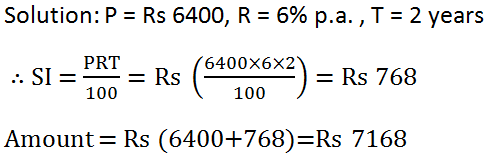# RS Aggarwal Class 7 Math Twelfth Chapter Simple Interest Exercise 12A Solution

## EXERCISE 12A

### Find the simple interest and the amount when:

(1) Principal = Rs 6400, Rate = 6% p.a. and time = 2 years.(2) Principal = Rs 2650, Rate = 8% p.a. and time = 2 ½ years.(3) Principal = Rs 1500, rate = 12% p.a. and time = 3 years 3 months.(4) Principal = Rs 9600, rate = 7 ½ % p.a. and time = 5 months.(5) Principal = Rs 5000, rate = 9% p.a. and time = 146 days.### Find the time when:

(6) Principal = Rs 6400, SI = Rs 1152 and rate = 6% p.a.(7) Principal = Rs 9540, SI = Rs 1908 and rate = 8% p.a.(8) Principal = Rs 5000, amount = Rs 6450 and rate = 12% p.a.### Find the rate when:

(9) Principal = Rs 8250, SI = Rs 1100 and time = 2 years.(10) Principal = Rs 5200, SI = 975 and time = 2 ½ years.(11) Principal = Rs 3560, amount = Rs 4521.20 and time = 3 years.(12) Shanta borrowed Rs 6000 from the State Bank of India for 3 years 8 months at 12% per annum. What amount will clear off her debt?(13) Hari borrowed Rs 12600 from a moneylender at 15% per annum simple interest. After 3 years, he paid Rs 7070 and gave a goat to clear off debt. What is the cost of the goat?(14) The simple interest on a certain sum for 3 years at 10% per annum is Rs 829.50. Find the sum.(15) A sum when reckoned at 7 ½ % per annum amounts to Rs 3920 in 3 years. Find the sum.(16) A sum of money put at 11% per annum amounts to Rs 4491 in 2 years. What will it amount to in 3 years at the same rate?(17) A sum of invested at 8% per annum amounts to Rs 12122 in 2 years. What will it amount to in 3 years at the same rate?(18) At what rate per cent per annum will Rs 3600 amount to 4734 in 3 ½ years?(19)  If Rs 640 amounts to Rs 768 in 2 years 6 months, what will Rs 850 amount to in 3 years at the same rate per cent per annum?(20) In what time will Rs 5600 amount to Rs 6720 at 8% per annum?(21) A sum of money becomes 8/5 of itself in 5 years at a certain rate of simple interest. Find the rate of interest.(22) A sum of money lent at simple interest amounts to Rs 4745 in 3 years. Find the sum and the rate per cent per annum.

(23) A sum of money lent at simple interest amounts to Rs 4745 in 3 years and to Rs 5475 in 5 years. Find the sum and the rate per cent per annum.(24) Divide Rs 3000 into two parts such that the simple interest on the first aprt for 4 years at 8% per annum is equal to the simple interest on the second part for 2 years at 9% per annum.Hence, the first part = Rs 1080 and Second part = Rs (300 – 1080) = Rs 1920.

(25) Divide Rs 3600 into two parts such that if one part be lent at 9% per annum and the other 10% per annum, the total annual income is Rs 333.Hence, the first part is Rs 2700 and second part is Rs (3600 – 2700) = Rs 900.

For more exercise solutions, Click Below –

Updated: May 30, 2022 — 1:33 pm